# PSAT Math : Negative Numbers

## Example Questions

2 Next →

### Example Question #26 : Integers

Given that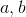are both integers,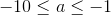, and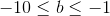, which of the following is correct about the sign of the expression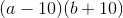?

The expression must be negative.

The expression can be positive, negative, or zero.

The expression must be negative or zero.

The expression must be positive.

The expression must be positive or zero.

The expression must be negative or zero.

Explanation:

If, then we know thatis any number between or equal to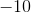and. Thereforemust be a negative number.

Also, if, then we know thatis any number between or equal toand. Thereforemust be a negative number.

Now looking the expressionwe can find the sign of each component in the expression.

Sinceis negative, we know that a negative number minus another number is still a negative number.

Therefore,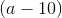is a negative number.

Sinceis between or equal toandwe can plug in these end values in to determine the sign of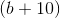.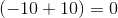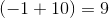Therefore,is either zero or a positive number.

Now to find the sign of the expression we look at the product of the two components. The product of a negative number and a positive number is a negative number; the product of a negative number and zero is zero. Therefore, the correct choice is thatis negative or zero.

### Example Question #21 : Integers

Find the product.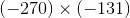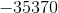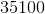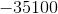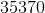Explanation:

When multiplying together two negatives, our value for the product become positive.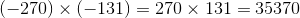### Example Question #28 : Integers

Find the product.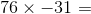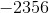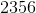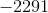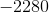Explanation:

Since we have one positive and one negative multiple, the resulting product must be negative.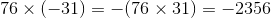### Example Question #1 : Negative Numbers

If x is a negative integer, what else must be a negative integer?

x² – x

x – x

x – (–x)

x – (–x)

Explanation:

By choosing a random negative number, for example: –4, we can input the number into each choice and see if we come out with another negative number.  When we put –4 in for x, we would have –4 – (–(–4)) or –4 – 4, which is –8.  Plugging in the other options gives a positive answer.  You can try other negative numbers, if needed, to confirm this still works.

### Example Question #1 : How To Add / Subtract / Multiply / Divide Negative Numbers

–7 – 7= x

–7 – (–7) = y

what are x and y, respectively

x = –14, y = 14

x = 0, y = 0

y = 0, x = 14

x = –14, y = 0

x = 14, y = –14

x = –14, y = 0

Explanation:

x: –7 – 7= –7 + –7 = –14

y: –7 – (–7) = –7 + 7 = 0

when subtracting a negative number, turn it into an addition problem

### Example Question #241 : Arithmetic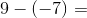15

13

2

5

16

16

Explanation:

Subtracting a negative number is just like adding its absolute value.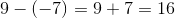2 Next →

### All PSAT Math Resources